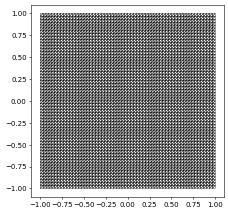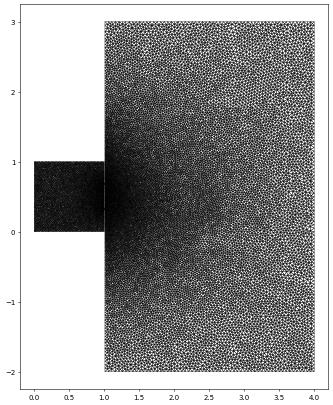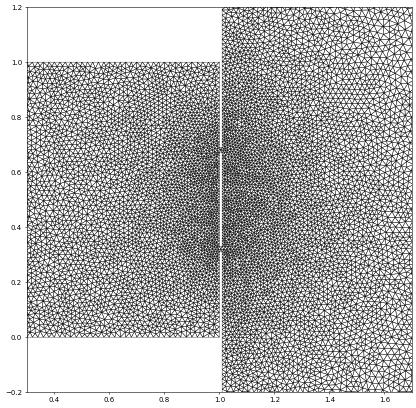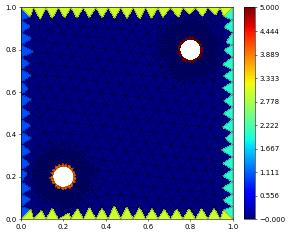# Examples of grid construction

Besides the already discussed construction of Cartesian grid and using dictionaries from general concepts it is also possible to read grid files using either the DGF or gmsh format (or other formats available through the meshio package).

:

import numpy
from matplotlib import pyplot
from dune.alugrid import aluSimplexGrid as leafGridView
from dune.fem.space import lagrange as solutionSpace
from dune.fem.scheme import galerkin as solutionScheme
from ufl import TestFunction, TrialFunction, SpatialCoordinate


## Example using the Dune Grid Format (DGF)

Please read the Dune Grid Format (DGF) for a detailed description of DGF. The format works for 2d or 3d and most DUNE grids can be constructed using a DGF file. The format allows easy description of boundary ids and parameters and is used as follows

:

domain2d = (reader.dgf, "triangle.dgf")

# specify dimgrid since it cannot be easily extracted from the dgf file
aluView = leafGridView(domain2d, dimgrid=2)
aluView.plot(figsize=(5,5))There is also a reader.gmsh option allowing previously stored gmsh files to be read. The grid is the one used in the wave equation example.

:

waveDomain = (reader.gmsh, "wave_tank.msh")
waveGrid = leafGridView(waveDomain, dimgrid=2)
waveGrid.plot(figure=pyplot.figure(figsize=(10,10)))## 3D example (using PyGmsh)

In this example we use pygmsh to construct a tetrahedral mesh and solve a simple Laplace problem. The following code is taken from the pygmsh homepage.

:

try:
import pygmsh
with pygmsh.geo.Geometry() as geom:
[ 0.0,  0.5, 0.0], [-0.1,  0.1, 0.0], [-0.5,  0.0, 0.0],
[-0.1, -0.1, 0.0], [ 0.0, -0.5, 0.0], [ 0.1, -0.1, 0.0],
[ 0.5,  0.0, 0.0], [ 0.1,  0.1, 0.0] ], mesh_size=0.05)
geom.twist(
poly,
translation_axis=[0, 0, 1], rotation_axis=[0, 0, 1], point_on_axis=[0, 0, 0],
angle=numpy.pi / 3,
)
mesh = geom.generate_mesh(verbose=False)

points, cells = mesh.points, mesh.cells_dict
domain3d = {"vertices":points.astype("float"), "simplices":cells["tetra"]}
except ImportError: # pygmsh not installed - use a simple cartesian domain
from dune.grid import cartesianDomain
domain3d = cartesianDomain([-0.25,-0.25,0],[0.25,0.25,1],[30,30,60])

gridView3d  = leafGridView(domain3d)
space3d = solutionSpace(gridView3d, order=1)

u = TrialFunction(space3d)
v = TestFunction(space3d)
x = SpatialCoordinate(space3d)
conditional(dot(x,x)<.01,100,0)*v*dx,
solver='cg')

uh3d = space3d.interpolate(,name="solution")
scheme3d.solve(target=uh3d)
# note: plotting with matplotlib not yet available for 3d grids
gridView3d.writeVTK('3dexample', pointdata=[uh3d],
outputType=OutputType.appendedraw)## Converting gmsh to DGF

Using the above convenient way of generating grids in Python we can then store those as DGF and add boundary ids for description of more complicated boundaries or parameters for vertices or cells. Use gmsh2DGF from gmsh2dgf to convert the above points and cells

:

from gmsh2dgf import gmsh2DGF
bndDomain = {1:"-0.6 -0.6 -0.1  0.6 0.6 0.0", # lower bnd gets id 1
2:"-0.6 -0.6  1.0  0.6 0.6 1.1", # upper bnd gets id 2
3: "default"} # all other boundary faces get id 3
dgf = gmsh2DGF(points, cells, bndDomain=bndDomain)
with open ("3dmesh.dgf", "w") as file:
file.write(dgf)


## Using meshio to read a gmsh file

We will use the meshio package to read a gmsh file, extract the points and cells and use that to construct a Dune grid. The final part is then quite similar to the above. We use the 2D grid from the wave equation test case. Note that this is a 2D grid but the points are returned as 3D points so we need to remove the final columns containing only zeros. We have already seen this grid above so let’s zoom in to the ‘slit’ region…

:

try:
import numpy as np
import meshio
points = np.delete( mesh.points, 2, 1) # remove the z component from the points
cells = mesh.cells_dict
waveDomain = {"vertices":points.astype("float"), "simplices":cells["triangle"]}
waveGrid = leafGridView(waveDomain)
waveGrid.plot(figure=pyplot.figure(figsize=(10,10)),xlim=[0.3,1.7],ylim=[-0.2,1.2])
except ImportError:
print("This example requires the meshio package which can be installed using pip.")Here is a second example where we want to read a gmsh containing quadrilateral elements. Note that the vertices of the cells are ordered counterclockwise which is not identical to the ordering required by the Dune reference element. The last two vertices need to be exchanged:

:

if meshio:
from dune.alugrid import aluCubeGrid
points2d = np.delete(mesh.points,2,1)
cells[:, [2, 3]] = cells[:, [3, 2]]
domain = {"vertices":points2d, "cubes":cells}## 1D example

It’s also possible to create grids in 1D

:

from dune.grid import onedGrid
v = [numpy.log(i) for i in range(1,100)]
e = [(i,i+1) for i in range(1,len(v))]
g = onedGrid(constructor={"vertices":v, "simplices":e})
s = solutionSpace(g)
x = SpatialCoordinate(s)
u = s.interpolate(sin(x), name="u")
u.plot()pyplot can very easily be used in 1d: pyplot.plot(g.tessellate(), u.pointData(), ‘-p’)

This page was generated from the notebook othergrids_nb.ipynb and is part of the tutorial for the dune-fem python bindings